# Year 11 Physics: Electricity and Magnetism Practice Questions

In this post, we share 10 Year 11 Physics: Electricity and Magnetism questions for you to test your skills on.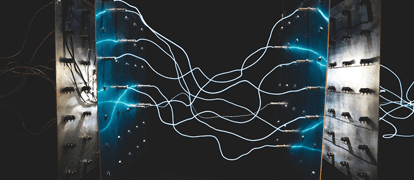## 2019 Year 11 Physics: Electricity and Magnetism Practice Questions

Are you fully charged and ready to go for your Yearly exams? Well, in this post, we’ve given you ten challenging electricity and magnetism practice questions to see how well you’ve attracted all of that Year 11 physics knowledge.

## Electricity and Magnetism Practice Questions

### Question 1

A metal rod with a negative charge is placed near a neutral metal conductor: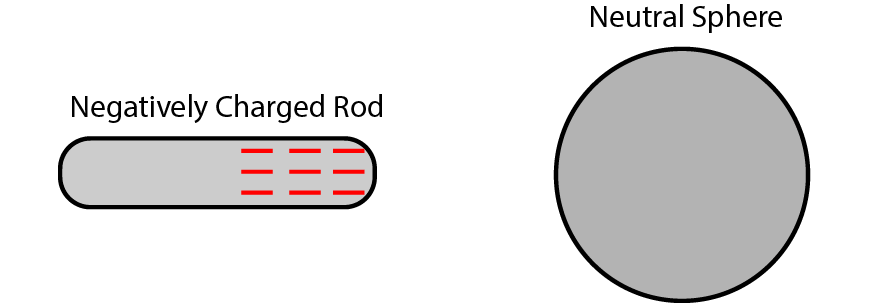a) Define the term ‘polarisation’ with respect to electric charge.

b) Show the charge distribution in the neutral sphere.

c) Outline a procedure whereby the sphere gains a negative charge.

d) Outline a procedure whereby the sphere gains a positive charge.

### Question 2

Draw the electric or magnetic field lines in the following diagrams: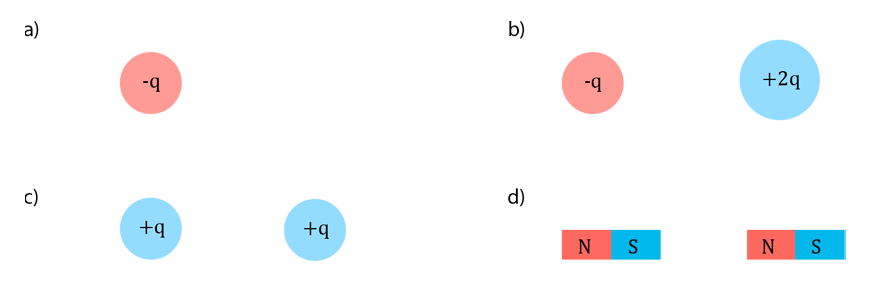### Question 3

Calculate the force on a test charge of $$+0.5\text{ }\mu\text{C}$$ in the following cases:

a) $$0.3\text{ m}$$ away from a $$+2.2\text{ }\mu\text{C}$$ charge.

b) Within an electric field of strength $$15\text{ Vm}^{-1}$$ upwards.

c) In the diagram below:### Question 4

A scientist is examining the motion of individual electrons in a particle accelerator. The electrons in question have a velocity of $$900,000\text{ ms}^{-1}$$.

a) Calculate the work on each electron required to achieve this speed, if the electrons began at rest.

b) Determine the voltage required to accelerate the electrons to this speed.

c) $$2.5 \times 10^{20}$$ electrons pass by a single point every second. Calculate the magnitude of the current.

d) Calculate the minimum power required to achieve the current in (c).

### Question 5

A voltage is applied across two parallel plates as in the diagram below: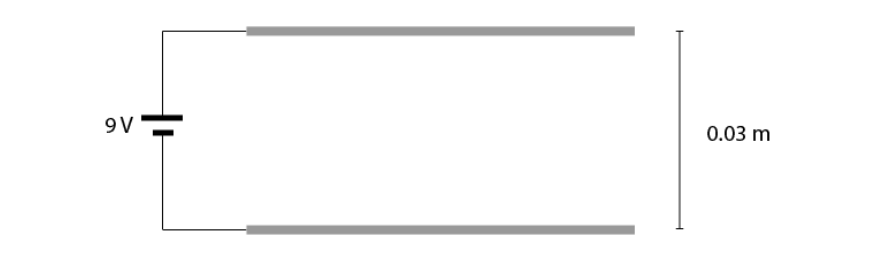a) Draw the electric field between the plates and indicate the strength of the electric field.

b) A charge of $$-100\text{ }\mu\text{C}$$ is suspended motionless between the plates. Calculate its mass.

c) Calculate the work done by the electric field on the $$-100\text{ }\mu\text{C}$$ charge if it moves down by $$1\text{ cm}$$.

### Question 6

An electric field has been set up between two parallel plates as shown below: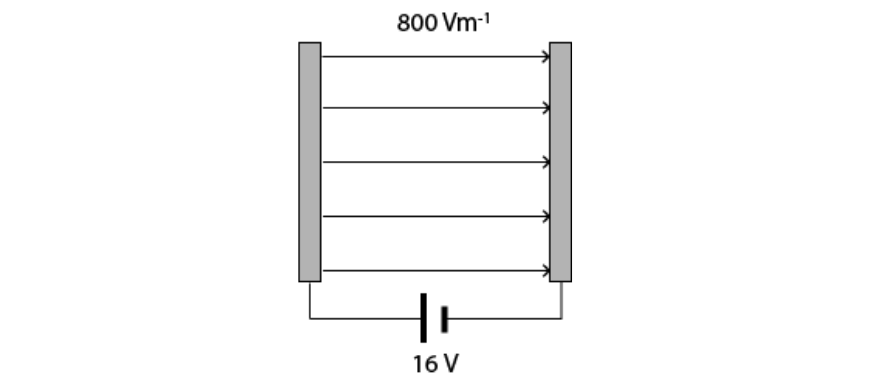a) What is the distance between the parallel plates?

b) Draw on the diagram an equipotential line corresponding to $$4\text{ V}$$ above the negative plate.

c) A negative charge is moved upwards along the $$4\text{ V}$$ equipotential line from (b). Outline the amount of work done by the electric field as the charge moves from one side of the equipotential line to the other and justify your answer.

### Question 7

A student was investigating Ohm’s law by measuring the voltage drop across a resistor as a function of current. Their recorded results are below:

 Current (Amps) Voltage (Volts) 0.2 2.13 0.4 4.29 0.5 5.20 0.6 6.33 0.7 7.38

a) By plotting the results on the graph below, determine if the resistor is ohmic.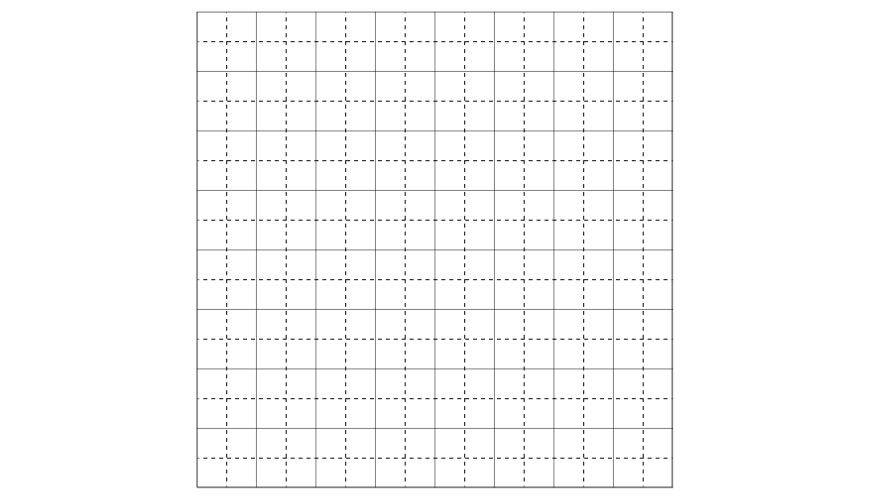b) Determine the resistance of the resistor for a current of $$0.3 \text{ A}$$.

### Question 8

A student constructs a circuit with an ammeter as shown below: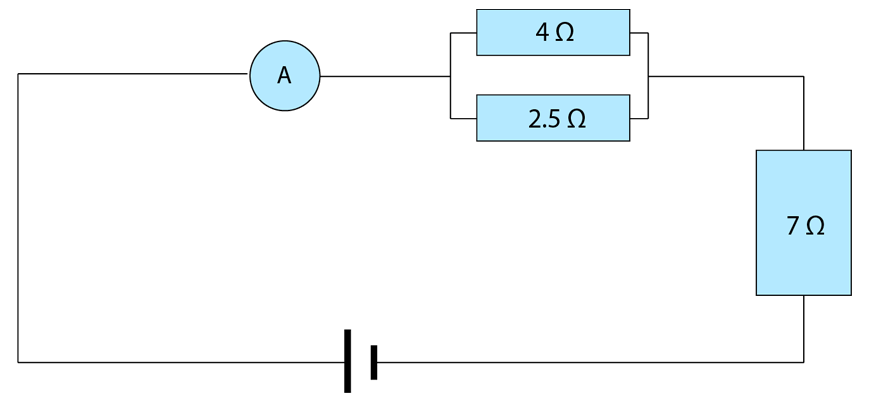a) Determine the total resistance of the circuit.

b) If the reading on the ammeter is $$1.5\text{ A}$$, calculate the voltage provided by the battery.

c) Determine the voltage drop across each of the resistors.

### Question 9

Consider the circuit below: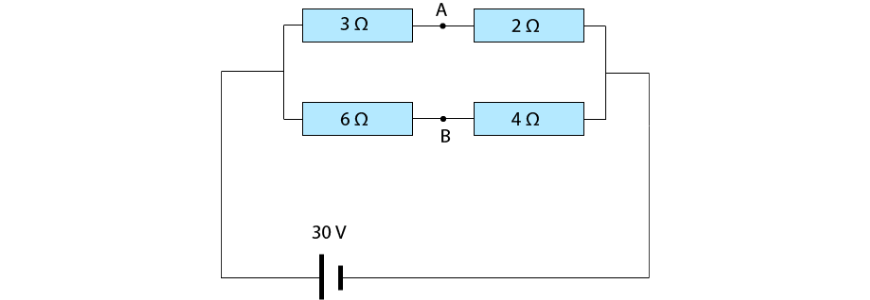a) Determine the total current in the circuit.

b) Determine the voltage drop across each resistor.

c) Calculate the power dissipated by each resistor.

d) Explain what would happen if a wire was connected between points A and B on the circuit [Hint: calculate the potential at points A and B].

### Question 10

A solenoid is an electrical device that can be used to generate a magnetic field, consisting of coils of wire wrapped around a core: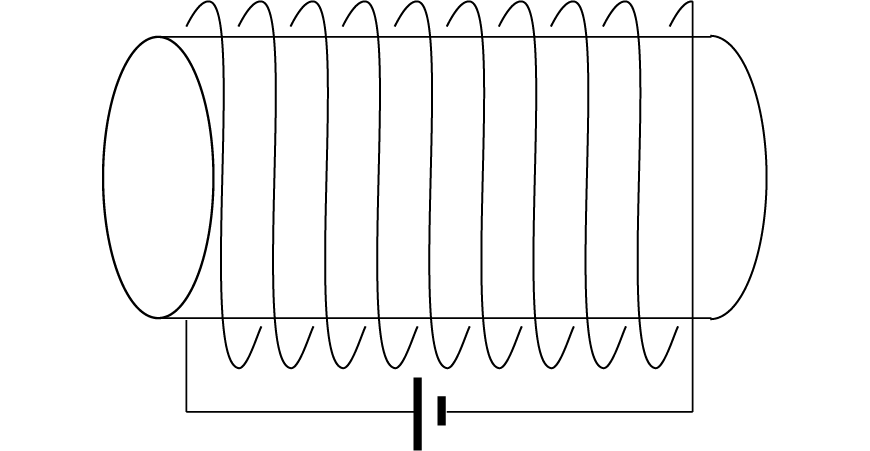The solenoid in the above diagram has $$10$$ turns, and is $$20 \text{ cm}$$ long.

a) Draw the magnetic field that will be generated by the solenoid. Label the north and south poles that are formed.

b) Calculate the strength of the magnetic field generated by a current of $$3.5 \text{ A}$$ running through the solenoid. Assume that there is no material in the core.

c) Outline how the magnetic field would change if a ferromagnetic material was added to the core.

## Need more practice with Electricity and Magnetism?

Get ahead of your peers with Year 11 Holiday Accelerated Courses. With advanced completion of contents before it’s taught at school, you’ll be better prepared for your school assessments.

Book your spot in our Year 11 Accelerated Physics today and join over 4500 students who already have a head start## Solutions

Question 1:

a) Polarisation refers to a separation of charge whereby one end of an object has a net positive charge and the other end has a net negative charge.

b)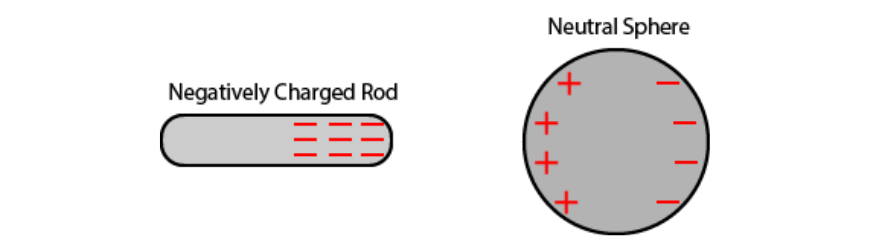c) Charging by contact: if the negatively charged rod touches the sphere some negative charge will be transferred onto the sphere, giving the sphere a net negative charge.

d) Charging by induction: The negatively charged rod is held close to the sphere but does not make contact. The sphere is instead connected to the ground with a conductor. Electrons move from the sphere to the ground, leaving the sphere with a net positive charge.

Question 2:

a)b)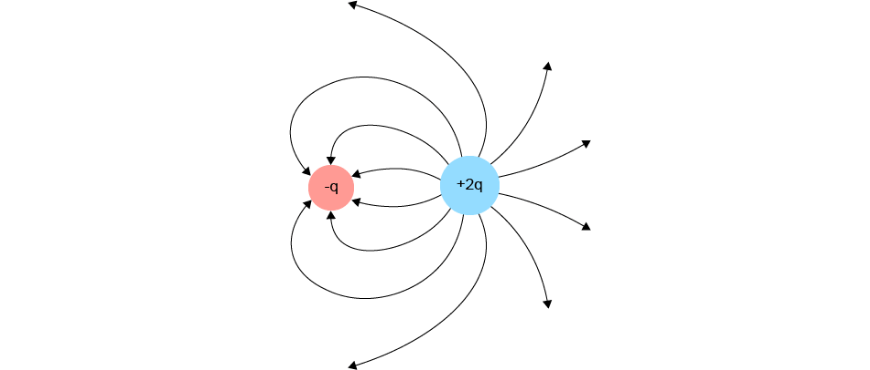c)d)Question 3:

a) $$0.11 \text{ N}$$ repulsive

b) $$7.5 \times 10^{-6} \text{ N}$$ upwards

c) $$1.48 \times 10^{-4} \text{ N}$$ to the right

Question 4:

a) $$3.69 \times 10^{-19} \text{ J}$$

b) $$2.3 \text{ V}$$

c) $$40 \text{ A}$$

d) $$92 \text{ W}$$

Question 5:

a)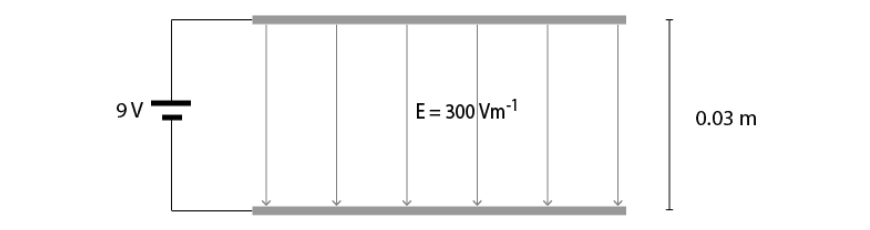b) $$3.06 \times 10^{-3}\text{ kg}$$

c) $$-3 \times 10^{-4}\text{ J}$$

Question 6:

a) $$0.02 \text{ m}$$

b)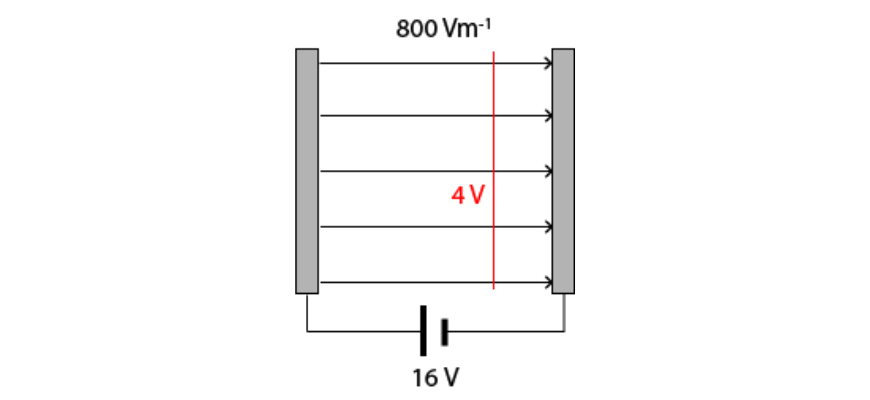c) Zero work is required to move along an equipotential. Equipotential surfaces are perpendicular to the field, and hence to the electric force a charge would experience. Thus the angle between the displacement and the force from the electric field is $$90^\circ$$ and the work done by the electric field is $$W = F s \text{ cos }\theta = F s \text{ cos }90^\circ = 0$$.

Question 7:

a)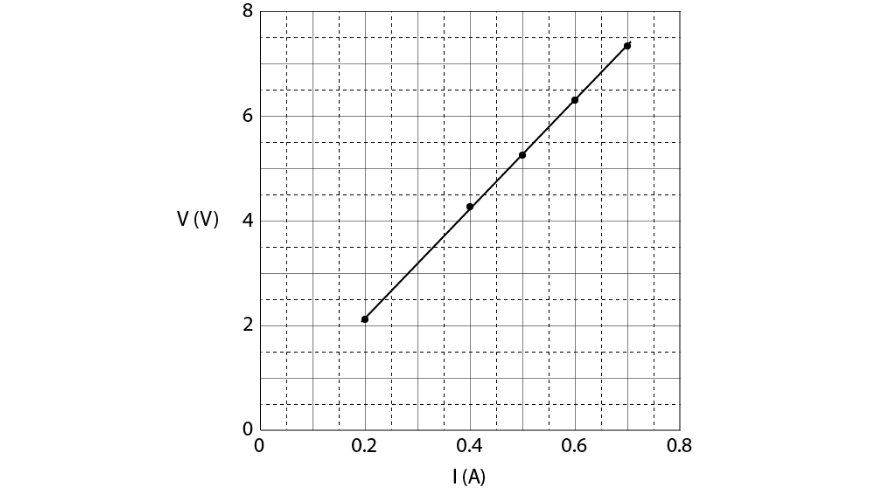The resistor is ohmic as voltage and current are proportional, indicated by the graph being a straight line.

b) $$10.5 \text{ }\Omega$$, resistance should be obtained from the gradient of the line of best fit (and is constant, independent of current).

Question 8:

a) $$8.54 \text{ }\Omega$$

b) $$12.8 \text{ V}$$

c) $$2.3 \text{ V}$$ for the  $$4.2 \text{ }\Omega$$ resistor.

$$2.3 \text{ V}$$ for the  $$2.5 \text{ }\Omega$$ resistor.

$$10.5 \text{ V}$$ for the  $$7 \text{ }\Omega$$ resistor.

Question 9:

a) $$9 \text{ A}$$

b) $$18 \text{ V}$$ for the $$3 \text{ }\Omega$$ resistor.

$$12 \text{ V}$$ for the $$2 \text{ }\Omega$$ resistor.

$$18 \text{ V}$$ for the $$6 \text{ }\Omega$$ resistor.

$$12 \text{ V}$$ for the  $$4 \text{ }\Omega$$ resistor.

c) $$108 \text{ W}$$ for the $$3 \text{ }\Omega$$ resistor.

$$72 \text{ W}$$ for the $$2 \text{ }\Omega$$ resistor.

$$54 \text{ W}$$ for the $$6 \text{ }\Omega$$ resistor.

$$36 \text{ W}$$ for the  $$4 \text{ }\Omega$$ resistor.

d) Nothing would happen. The potential at point A is $$12 \text{ V}$$ and the potential at point B is $$12 \text{ V}$$. Since there is no potential difference between points A and B, no current will flow if they are connected.

Question 10:

a)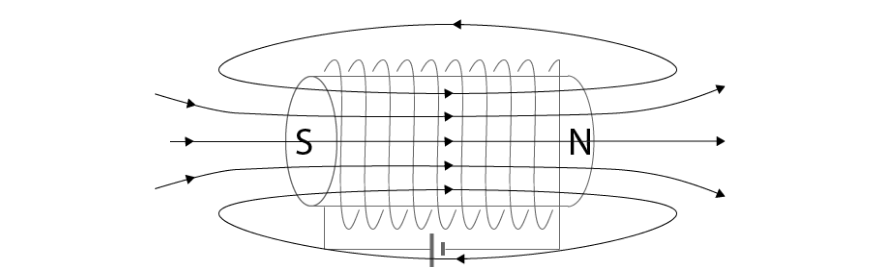b) $$B = 2.2 \times 10^{-4}\text{ T}$$

c) The magnetic field strength would greatly increase.